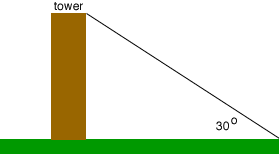Quandaries and Queries Hello my name is Augustin, i am a student, and i am going to ask a question in trig. secondary. If the distance of a person from a tower is 100 m and the angle subtended by the top of the tower with the ground is 30 degrees, what is the height of the tower in meters?   Distance from the tower to the person is given as 100m and we have to find the height of the tower. But it is given that "the angle subtended by the top of the tower with the ground is 30 degrees".  My math teacher said that we have to take that the angle subtended by the top of the tower with the person to be 30 degrees.  I can't get this.  I want to know why we are taking the angle from the top of the tower to the person as 30 degrees since it is given that 30 degrees = angle subtended by the top of the tower to the ground. Please explain this.  Thank u. Augustin :) Hi Augustin, I find this a strangely worded question also. Fist the word subtend means to lie opposite. For example the hypotenuse of a right triangle lies opposite to the right angle, so you might say that a hypotenuse subtends an angle of 90 degrees. In your problem I would have said that "the tower subtends an angle of 30 degrees...", so I see a triangle with one side the tower and the angle opposite the tower being 30 degrees.So where is this point on the ground where the 30 degree angle is formed? If you are to find the height of the tower from this diagram you need to know the distance, along the ground, from the base of the tower to this point where the 30 degree angle sits. The only distance you are given is that there is a person, 100 meters from the tower hence the question must mean that the point where the 30 degree angle sits is the point where the person is standing. I hope this helps. Harley Go to Math Central